# The WDD test with different pre/post time periods

Eric Piza asked the other day if my and Jerry’s WDD test can be used when the pre/post time periods are different. The answer is yes out of the box, the identification strategy does not rely on equality of time periods. So for example, say we had two years pre and one year post data, and the crime counts in treated/control looked like this:

``````         Pre  Post
Treated   80    20
Control  100    50``````

So then our difference-in-difference Poisson estimate of the treatment effect would be:

``(20 - 80) - (50 - 100) =  -10``

What the parallel trends assumption means here is that since you saw a decrease in 50 crimes in the control area, you would expect a decrease of 50 crimes in the treated area as well. The variance of this estimate is then `20 + 80 + 50 + 100 = 250`, and so the standard error is `sqrt(250) ~ 15.8`. So this is not a statistically significant effect.

It is hard to interpret this effect size though, since it is not a standard unit of time comparison. Also the variance of the estimate will be larger if you have a longer pre time period, which is the opposite of what you want. We can actually amend the statistic though to be a per-unit-time comparison, which will reduce the variance of the estimate. It ends up being similar to my prior post on adding Harm Weights to the WDD, you can’t just pipe in the per unit time estimates in the spreadsheet I shared, but I will show here how to incorporate them into the estimator (and share some python code to show the estimator behaves as expected in simulations).

So again with a pre-time period of 2 years, and post of 1 year, we could do the prior table as per year estimates.

``````         Pre  Post
Treated   40    20
Control   50    50``````

And here our estimate of the crime reduction effect is different:

``(20 - 40) - (50 - 50) =  -20``

So with a Poisson variable with a mean of 100, the variance of that variable is also 100. So here we are dividing that 100 by a constant 2 – this changes the variance to 100/(2^2). (`Var(X*a) = a^2*Var(X)` where `X` is a random variable and `a` is a constant.) The post variables are simply divided by one, so does not change their variance. So to carry this forward to our standard error estimate, we would calculate (edit, had some errors in this part, should be using the original counts, not the average counts, but my later python code was correct, so the simulation part is OK):

``20/1 + 80/4 + 50/1 + 100/4 = 115``

So you can see that our variance estimate here is much smaller, and that the standard error is `sqrt(115) ~ 10.7`. So here the reduction is not quite a statistically significant reduction in crimes at the 0.05 level. A 95% confidence interval would be `-20 +/- 2*10.7 ~ [+1, -41]`. Here the WDD estimate is easier to interpret as well, and that confidence interval corresponds to a per year estimate of somewhere between +1 and -41 crimes.

Below I share some python code to conduct simulations similar to the original WDD paper. This code will then establish the estimator has the null distribution as expected (when there are no changes it really is a standard normal distribution) and that the confidence intervals have coverage like you would expect.

# Python Simulation Code

For set up, I import the libraries I need (stat distributions, numpy and pandas). I am not going to go into detail into the functions, but it allows you to generate simulated distributions in various ways to conduct analysis of the properties of my time weighted estimator I have specified above.

``````'''
WDD Simulation with differing time periods
Andy Wheeler
'''

import pandas as pd
import numpy as np
from scipy.stats import norm
from scipy.stats import poisson
from scipy.stats import uniform

#This works for the scipy functions
np.random.seed(seed=10)

# A function to generate the WDD estimate for simulated data
def wdd_sim(treat0,treat1,cont0,cont1,pre,post):
tr_cr_0 = poisson.rvs(mu = treat0, size=int(pre)).sum()
co_cr_0 = poisson.rvs(mu = cont0, size=int(pre)).sum()
tr_cr_1 = poisson.rvs(mu = treat1, size=int(post)).sum()
co_cr_1 = poisson.rvs(mu = cont1, size=int(post)).sum()
est = ( tr_cr_1/post - tr_cr_0/pre ) - ( co_cr_1/post - co_cr_0/pre )
post2 = (1/post)**2
pre2 = (1/pre)**2
var_est = tr_cr_0*pre2 + tr_cr_1*post2 + co_cr_0*pre2 + co_cr_1*post2
true_val = ( treat1 - treat0 ) - ( cont1 - cont0 )
z_score = est / np.sqrt(var_est)
return (est, var_est, true_val, z_score)

def make_data(n, treat0, treat1, cont0, cont1, pre, post):
base = pd.DataFrame( range(n), columns=['index'])
base['treat0'] = treat0
if treat1 is not None:
base['treat1'] = treat1
else:
base['treat1'] = base['treat0']
if cont0 is not None:
base['cont0'] = cont0
else:
base['cont0'] = base['treat0']
if cont1 is not None:
base['cont1'] = cont1
else:
base['cont1'] = base['cont0']
base.drop(columns='index',inplace=True)
base['pre'] = pre
base['post'] = post
sim_vals = base.apply(lambda x: wdd_sim(**x), axis=1, result_type='expand')
sim_vals.columns = ['est','var_est','true_val','z_score']
return pd.concat([base,sim_vals], axis=1)``````

So for a first example, this code generates treatment/control areas with a Poisson mean of 5 in both the pre/post time periods. But, the pre time period is 4 units of time, and the post time period is only 1 unit. So this means there is no change, and the Z score estimator should on average have a 0 estimate and a standard deviation of 1. I do 10,000 simulations to keep it going a bit faster, but you can up that if you want.

``````# No change, with baseline of 5 crimes per unit time
sim_dat = make_data(10000, 5, 5, 5, 5, 4, 1)
sim_dat['z_score'].describe()``````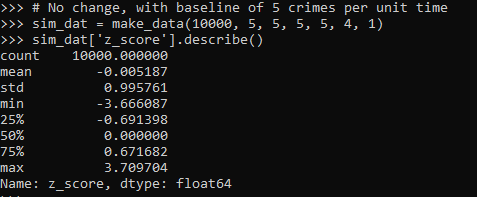So here we can see these 10k simulated Poisson data have a mean z-score of 0 and a standard deviation of 1, right like we expected.

So I haven’t extensively tested, but if you have average crime counts well under 5, I would be a bit hesitant to use this estimator. (So you either need larger area aggregations or larger time aggregations.) Although you could do simulations on your own to see how it holds up.

The way I wrote the functions you can also pass in random variables as well, so here is an example with again no change, but the baseline varies uniformily from 5 to 100. And here also the pre time periods are 6, and the post time period is again just 1.

``````# Can pass in random functions instead of constant values
sim_n = 10000
tf = uniform.rvs(loc=5, scale=100, size=sim_n)

sim_dat2 = make_data(sim_n, tf, None, None, None, 6, 1)
sim_dat2['z_score'].describe()``````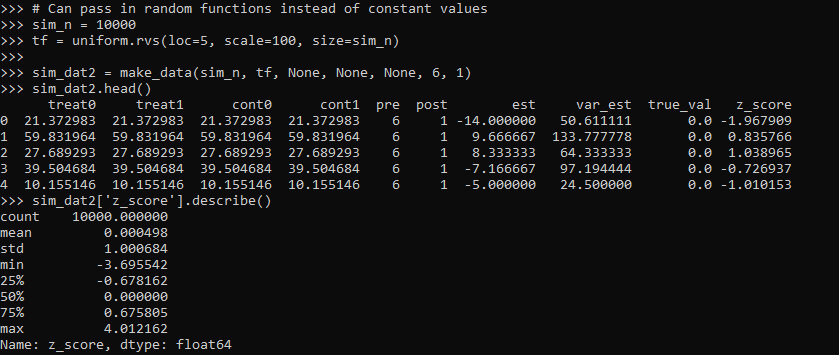So you can see the base simulated dataset pre/post always has the same means, but instead of being a set of constant 5’s, it changes for each row (simulation) in the dataset. And again the null distribution is right on the money with a mean of 0 and standard deviation of 1.

So those are examples of the null distribution of no changes in the time weighted estimator. This establishes that the false positive alpha rates are as you would expect. E.g. if you use the usual p-value < 0.05, if the differences are really 0 you only have a false positive reject the null 5 times out of 100.

But we also want to establish that when there is a difference, the estimator is not biased and that the variance estimates are correct. For the later part looking at the coverage rates of the confidence intervals is one way to do that. So here I show that with my hypothetical example in the intro part of this blog, the 95% and 90% confidence interval coverage rates are exactly as they should be. And the z-score estimate is right about where it should be as well.

``````# Lets look at the coverage rate for a decline from 40 to 20
def cover(data, ci=0.95):
mult = (1 - ci)/2
nv = norm.ppf(1 - mult)
dif = nv*np.sqrt( data['var_est'] )
low = data['est'] - dif
high = data['est'] + dif
cover = ( data['true_val'] > low) & ( data['true_val'] < high )
return cover

sim_dat3 = make_data(sim_n, 40, 20, 50, 50, 2, 1)

# This should be centered on 2
sim_dat3['z_score'].describe()

# Should be ~ 0.9
co_90 = cover(sim_dat3, ci=0.9)
co_90.mean()

# Should be ~ 0.95
co_95 = cover(sim_dat3, ci=0.95)
co_95.mean()``````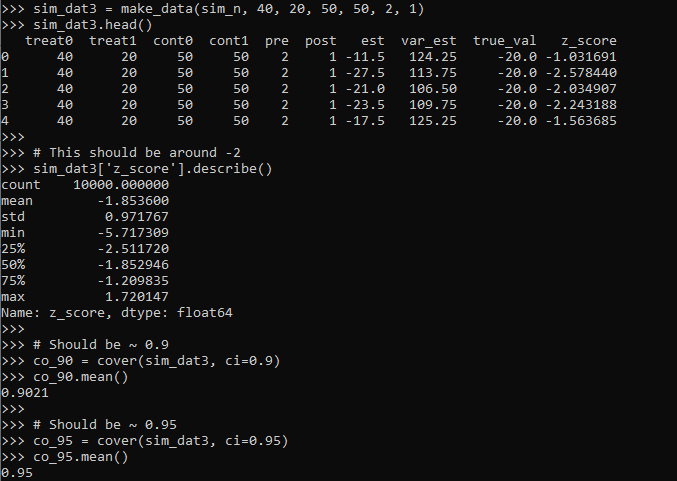So you can see the coverage is right on the money. The estimator is slightly biased downward in this simulation (should get a z-score on average around -2, but here the mean is -1.85). But it is good enough IMO to not worry about much in this situation.

Again, the original estimator without weighted for time is fine, if we do the same motions without doing weighting for different time periods, the coverage is still all fine and dandy.

``````# Note you can do the same coverage estimate without time weighted
sim_dat4 = make_data(sim_n, 80, 20, 100, 50, 1, 1)

# This should be around -0.6
sim_dat4['z_score'].describe()

co_90w = cover(sim_dat4, ci=0.9)
co_90w.mean()

co_95w = cover(sim_dat4, ci=0.95)
co_95w.mean()``````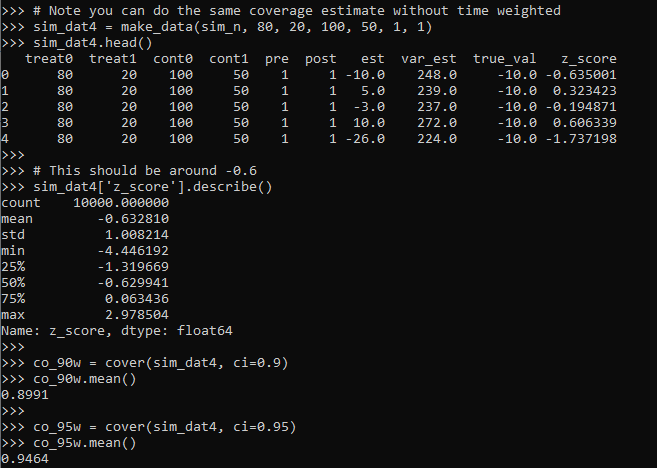So you can see again coverage is right on the money, and the z-score estimator actually has less bias than the time weighted one, it is right on the money as expected.

So why would you prefer the time weighted estimator if it shows more bias? It is because it has a lower variance, this code shows the length of the confidence intervals in the simulations.

``````# Does it make a difference?
def len_ci(data, ci=0.95):
mult = (1 - ci)/2
nv = norm.ppf(1 - mult)
dif = nv*np.sqrt( data['var_est'] )
low = data['est'] - dif
high = data['est'] + dif
return high - low

len4 = len_ci(sim_dat4)
len4.describe()

len3 = len_ci(sim_dat3)
len3.describe()``````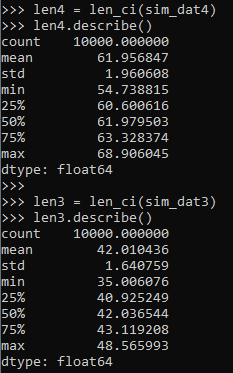So you can see here that the non-time weighted estimator tends to have a confidence interval with a length of 62, whereas the time weighted estimator has a confidence interval on average of 42.

So above establishes that the time weighted estimator behaves as you would expect. You can also use this code to conduct some potential power analyses. So for the time weighted estimator we show, even though the reduction is around 50% in the treated area (going from 40 to 20), the power is not great, around 60%.

``````# Example power analyses, ONE TAILED
def reject_rate(data, alpha=0.05):
p_vals = norm.cdf(data['z_score'])
return p_vals < alpha

r3 = reject_rate(sim_dat3)
r3.mean()``````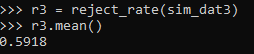So this means if you did this experiment in real life and it was that effective, you would still fail to reject the null of no differences 2/5 times.

But what if we say we will get more historical data? So 4 years back instead of just 2? How does that impact our power estimates?

``````# How about with more historical data
sim_dat5 = make_data(sim_n, 40, 20, 50, 50, 4, 1)
r5 = reject_rate(sim_dat5)
r5.mean()``````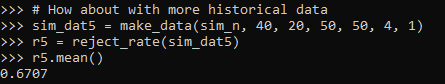The power goes up by alittle, to 0.67. The same is true if we up the post period to 4 time periods instead of 1:

``````# How about with more post data
sim_dat6 = make_data(sim_n, 40, 20, 50, 50, 4, 4)
r6 = reject_rate(sim_dat6)
r6.mean()``````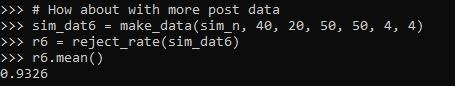So now in this example you have an over 90% power to detect a crime reduction, going from 40 to 20 per time period (where the control has an average of 50 crimes per time period), if you have 4 pre time periods and 4 post time periods.

# Future Stuff

So a few caveats with this. For one, you may think that since dividing per time period reduces the variance, why not divide by smaller time slivers. So instead of one year, why not divide by 365 days?

I have not studied extensively this property of the estimator. So I cannot say how it behaves with more/less time aggregation into smaller Poisson estimates. You will need to take that on yourself if you want to examine very fine time units and very small Poisson counts per unit time. Again I think a baseline rule of thumb that they should not be lower the 5 counts per unit time is the best advice I can give without doing simulations for your exact circumstances.

A second part is that with longer time periods comes the risk that the control areas are not as good. This is a problem intrinsic to synthetic control analysis as well (that I don’t believe anyone has a particular answer to). And I don’t have an answer either.

For the pre-time period, you can check the parallel trends assumption by simply plotting the two time series, they should be close to in step with one another. So that is not a big deal. But with the post time period, I think if you monitor long enough they will eventually depart from one another.

So I think it is best to set up a time period at the start you have committed to doing the experiment. And you can use the power analysis simulations like I showed to help you figure out that period. But it may be possible to extend this WDD estimate to continuously monitor an intervention (see here for example).